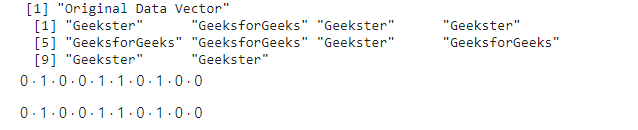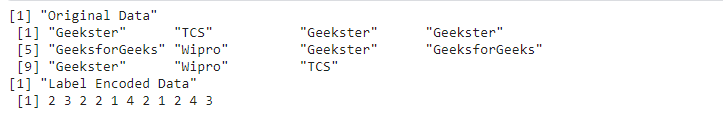Open in App
Not now

# Label Encoding in R programming

• Last Updated : 09 Oct, 2022

The data that has to be processed for performing manipulations and Analyses should be easily understood and well denoted. The computer finds it difficult to process strings and other objects when data training and predictions based on it have to be performed. Label encoding is a mechanism to assign numerical values to the string variables so that they are easily transformed and fed into various models. Therefore label encoders typically perform the conversion of categorical variables into integral values. Decoders perform the reverse operation.

## Label Encoding in R programming

Label encoders take as input a vector of categorical variables and convert it into numerical form. Initially, a vector is fed as input to the encoder.

To implement the Label Encoding in R Programming Language, we have two methods :

1. Using superml
2. Using factors()

Let’s discuss the method below:

## Using superml to Get Label Encoding in R programming

The superml package in R is designed to unify the model training process in R. It can be downloaded and installed into the working space using the following command :

`install.packages("superml")`

Initially, a new label encoder object is instantiated using LabelEncoder\$new(). The vector supplied as input is used for fitting the model. The transformation takes place using the fit_transform method, which performs the transformation. The final result is the numerical vector.

The following sequence of operations is performed :

• encoder\$fit(x)
• encoder\$fit_transform(x)
• encoder\$transform(x)

Arguments :

• x – The vector to be supplied
• In the following code snippet, there were 2 groups therefore, numerically a binary vector of 0s and 1s have been created.

After installing the superml library with the above mentioned command, we can now run the below code.

## R

 `x = ``c``(``"Geekster"``,``"GeeksforGeeks"``,``"Geekster"``,``"Geekster"``, ` `      ``"GeeksforGeeks"``,``"GeeksforGeeks"``,``"Geekster"``,``"GeeksforGeeks"``, ` `      ``"Geekster"``,``"Geekster"``) ` ` `  `print``(``"Original Data Vector"``) ` `print``(x ) ` ` `  `# create a label encoder object ` `encoder = LabelEncoder\$``new``() ` ` `  `# fitting the data over the x vector ` `encoder\$``fit``(x) ` ` `  `# transforming the data ` `encoder\$``fit_transform``(x) ` ` `  `# printing the transformed data ` `encoder\$``transform``(x)`

Output:## Using factors() to Get Label Encoding in R programming

The factors method in base R is used to transform the given data into categorical variables. The values are assigned to each of the variables. In case, we wish to use the numerical instances, we can simply use as.numeric() method for the conversion.

Syntax : factor(x)

Arguments : x – The vector to be encoded

In the following code, the data contained in the companies vector is first sorted lexicographically. The levels are then assigned to the values and mapped to integers beginning with 1. The word “GeeksForGeeks” is assigned 1 level, and all its occurrences are replaced with 1 in the final output.

## R

 `# creating a data vector ` `companies =  ``c``(``"Geekster"``,``"TCS"``,``"Geekster"``,``"Geekster"``, ` `               ``"GeeksforGeeks"``, ` `               ``"Wipro"``,``"Geekster"``, ` `               ``"GeeksforGeeks"``, ` `               ``"Geekster"``,``"Wipro"``,``"TCS"``) ` ` `  `# printing the original vector ` `print``(``"Original Data"``) ` `print``(companies) ` ` `  `# converting the data to factors ` `factors <- ``factor``(companies) ` ` `  `# converting data to label encoded values ` `print``(``"Label Encoded Data"``) ` ` `  `# printing the numeric equivalents of these vector values ` `print``(``as.numeric``(factors))`

Output :My Personal Notes arrow_drop_up
Related Articles